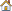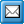﻿ Using Range Values
QPR Knowledge Base 2017.1

Using Range ValuesMetrics User's Guide > Using QPR Metrics > Ranges:

Using Range ValuesUsing Range ValuesRange values make it possible to compile values of different units.

For example, let's say that a top element Scorecard should be calculated with a formula that takes the average of element Customer and element Processes. Now let's say that Customer's value is \$12,000 and Processes' value is 17,5%. Calculating the average of these values would not correctly show the performance of the top element Scorecard. This is when range values become very useful.

Assume that the ranges and range values of these elements are as follows: Excellent = 10, Average = 5, and Poor =0.

So if Customer's value (\$12,000) falls into the range "Excellent", then its range value is 10.

And if Processes' value (17,5%) falls into the range "Average", then its range value is 5.

Therefore when the formula of the top element, Scorecard, calculates the average of the 2 elements, it gets 7.5, the average of the range values. So if it's upper limit value (e.g. Target value) was also calculated using range values and its values was calculated to be 8, then the Scorecard's value would fall into the Average (yellow) range. With this technique, the calculated result will correctly express the overall performance.